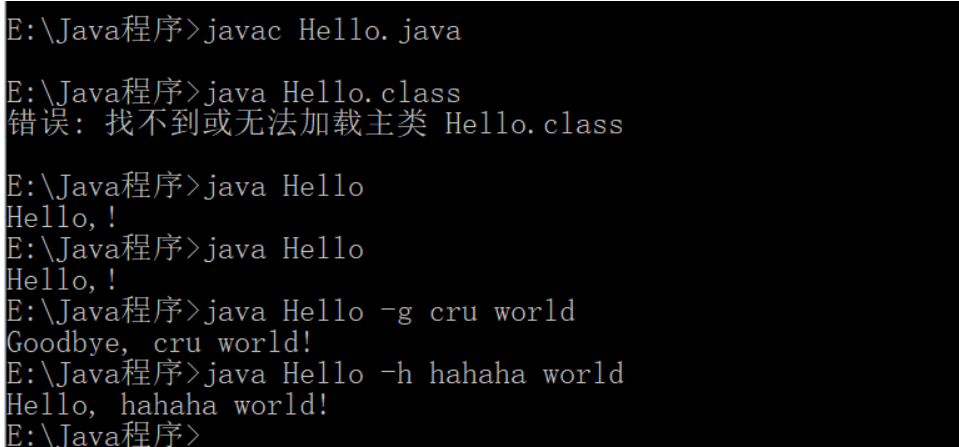• 有个问题，boolean 不是只能跟Ture或者false么，这样写一个数组，是单纯定义一个数组，还是把这个flags的数组已经定义成boolean 的一个值了 char[] chs = new char; for (int i = 0; i ; i++) { int index; do { ...
publicstaticchar[]generate(){//定义了一个方法从26个字母随机取出5个字母不能重复char[]letters={'A','B','C','D','E','F','G','H','I','J','K','L','M','N','O','P','Q','R','S','...public static char[] generate() {//定义了一个方法从26个字母随机取出5个字母不能重复char[] letters = { 'A', 'B', 'C', 'D', 'E', 'F', 'G', 'H', 'I', 'J','K', 'L', 'M', 'N', 'O', 'P', 'Q', 'R', 'S', 'T', 'U', 'V','W', 'X', 'Y', 'Z' };boolean[] flags = new boolean[letters.length];  //问题一：这句是定义一个boolean类型的数组吗？有个问题，boolean 不是只能跟Ture或者false么，这样写一个数组，是单纯定义一个数组，还是把这个flags的数组已经定义成boolean 的一个值了char[] chs = new char;for (int i = 0; i < chs.length; i++) {int index;do {index = (int) (Math.random() * (letters.length));} while (flags[index]);    // 问题二：while这句括号里的值第一次取值，比如为flags时，它是怎么判定为false出来的？还是说最初定义boolean[]flags数组时，就已经是false了，因为下面把取过值的数定义为ture了，会再次循环的。就是想知道此处如何判定为false出来的chs[i] = letters[index];flags[index] = true;}return chs;}展开
展开全文• java里面用boolean数组，100000100大小的boolean数组不超内存找球号(二)时间限制：1000ms | 内存限制：65535KB难度：5描述在某一国度里流行着一种游戏。游戏规则为：现有一堆球，每个球上都有一个整数编号i(0<=...
java里面用boolean数组，100000100大小的boolean数组不超内存找球号(二)时间限制：1000ms  |  内存限制：65535KB难度：5描述在某一国度里流行着一种游戏。游戏规则为：现有一堆球中，每个球上都有一个整数编号i(0<=i<=100000000),编号可重复，还有一个空箱子，现在有两种动作：一种是"ADD"，表示向空箱子里放m(0输入第一行有一个整数n(0随后有n行;每行可能出现如下的任意一种形式：第一种：一个字符串"ADD"，接着是一个整数m，随后有m个i；第二种：一个字符串"QUERY”，接着是一个整数M，随后有M个ki；输出输出每次询问的结果"YES"或"NO".样例输入2ADD 5 34 343 54 6 2QUERY 4 34 54 33 66样例输出YESYESNONO#include#include#include#includeusing namespace std;int main(){int n,i,m,p,j,y;char char1;vectorx;while(scanf("%d",&n)!=EOF){for(i=0;i{scanf("%s",char1);scanf("%d",&m);if(char1=='A'){for(j=0;j{scanf("%d",&p);x[p%10001].push_back(p);}}else{for(j=0;j{int flag=0;scanf("%d",&y);for(int k=0;k< x[y%10001].size();k++){if( x[y%10001][k]==y){ flag=1;printf("YES\n");break;}}if(flag==0)printf("NO\n");}}}}return 0;}java代码acimport java.util.Arrays;import java.util.Scanner;public class Main {public static void main(String[] args) {Scanner scanner = new Scanner(System.in);while (scanner.hasNext()) {int time = scanner.nextInt();boolean result[]=new boolean;Arrays.fill(result, false);while (time-- != 0) {String string=scanner.next();if(string.charAt(0)=='A'){int number=scanner.nextInt();for(int i=0;i{int x=scanner.nextInt();result[x]=true;}}else {int num=scanner.nextInt();while(num--!=0){int y=scanner.nextInt();if(result[y])System.out.println("YES");else {System.out.println("NO");}}}}}}}java用vector不过代码，在找原因import java.util.Scanner;import java.util.Vector;class result{Vector vector=new Vector();}public class Main {static result results[];public static void main(String[] args) {Scanner scanner = new Scanner(System.in);results=new result;for(int i=0;i<1005;i++)results[i]=new result();int time = scanner.nextInt();while (time--!=0) {String string = scanner.next();int number = scanner.nextInt();if (string.charAt(0) == 'A') {for (int i = 0; i < number; i++) {int x = scanner.nextInt();results[x%1001].vector.add(x);}} else {for (int j = 0; j < number; j++) {int y = scanner.nextInt();if(results[y%1001].vector.isEmpty()==true)System.out.println("NO");else{int flag = 1;for(int i=0;i{if(results[y%1001].vector.get(i)==y){System.out.println("YES");flag=0;break;}}if (flag == 1)System.out.println("NO");}}}}}}
展开全文• java里面用boolean数组，100000100大小的boolean数组不超内存 找球号(二） 时间限制：1000 ms | 内存限制：65535 KB 难度：5 描述在某一国度里流行着一种游戏。游戏规则为：现有一堆球，每个球上都...


java里面用boolean数组，100000100大小的boolean数组不超内存

找球号(二）
时间限制：1000 ms  |  内存限制：65535 KB
难度：5

描述在某一国度里流行着一种游戏。游戏规则为：现有一堆球中，每个球上都有一个整数编号i(0<=i<=100000000),编号可重复，还有一个空箱子，现在有两种动作：一种是"ADD"，表示向空箱子里放m(0<m<=100)个球，另一种是"QUERY”，表示说出M(0<M<=100)个随机整数ki(0<=ki<=100000100),分别判断编号为ki 的球是否在这个空箱子中（存在为"YES",否则为"NO"），先答出者为胜。现在有一个人想玩玩这个游戏，但他又很懒。他希望你能帮助他取得胜利。

输入第一行有一个整数n(0<n<=10000);
随后有n行;
每行可能出现如下的任意一种形式：
第一种：
一个字符串"ADD"，接着是一个整数m，随后有m个i；
第二种：
一个字符串"QUERY”，接着是一个整数M，随后有M个ki；
输出输出每次询问的结果"YES"或"NO".
样例输入
2
ADD 5 34 343 54 6 2
QUERY 4 34 54 33 66

样例输出YESYESNONO

#include<iostream>
#include<vector>
#include<cstdio>
#include<algorithm>
using namespace std;
int main()
{
int n,i,m,p,j,y;
char char1;
vector<int>x;
while(scanf("%d",&n)!=EOF)
{
for(i=0;i<n;i++)
{
scanf("%s",char1);
scanf("%d",&m);
if(char1=='A')
{
for(j=0;j<m;j++)
{
scanf("%d",&p);
x[p%10001].push_back(p);
}
}
else
{
for(j=0;j<m;j++)
{
int flag=0;
scanf("%d",&y);
for(int k=0;k< x[y%10001].size();k++)
{
if( x[y%10001][k]==y)
{ flag=1;printf("YES\n");break;}
}
if(flag==0)
printf("NO\n");
}
}
}
}
return 0;
}

java代码ac



import java.util.Arrays;
import java.util.Scanner;
public class Main {

public static void main(String[] args) {
Scanner scanner = new Scanner(System.in);
while (scanner.hasNext()) {
int time = scanner.nextInt();
boolean result[]=new boolean;
Arrays.fill(result, false);
while (time-- != 0) {
String string=scanner.next();
if(string.charAt(0)=='A')
{
int number=scanner.nextInt();
for(int i=0;i<number;i++)
{
int x=scanner.nextInt();
result[x]=true;
}
}
else {
int num=scanner.nextInt();
while(num--!=0)
{
int y=scanner.nextInt();
if(result[y])
System.out.println("YES");
else {
System.out.println("NO");
}
}
}
}

}
}
}


java用vector不过代码，在找原因
import java.util.Scanner;
import java.util.Vector;
class result
{
Vector<Integer> vector=new Vector<Integer>();
}
public class Main {

static result results[];
public static void main(String[] args) {
Scanner scanner = new Scanner(System.in);
results=new result;
for(int i=0;i<1005;i++)
results[i]=new result();
int time = scanner.nextInt();
while (time--!=0) {
String string = scanner.next();
int number = scanner.nextInt();
if (string.charAt(0) == 'A') {
for (int i = 0; i < number; i++) {
int x = scanner.nextInt();
results[x%1001].vector.add(x);
}
} else {

for (int j = 0; j < number; j++) {
int y = scanner.nextInt();
if(results[y%1001].vector.isEmpty()==true)
System.out.println("NO");
else{
int flag = 1;
for(int i=0;i<results[y%1001].vector.size();i++)
{
if(results[y%1001].vector.get(i)==y)
{
System.out.println("YES");
flag=0;break;
}
}
if (flag == 1)
System.out.println("NO");
}
}
}
}

}
}






展开全文vector query ini
• 3.10数组 ...在java中不要求数组长度是常量。 在创建一个数字数组时，所有元素都初始化为0。boolean数组的元素初始化为false。对象数组的元素初始化为null，这表示这些元素还未存放任何对象。 3.10.1 for e
3.10数组
数组是一种数据结构，用来存贮同一类型值得集合。
在声明数组变量时，需要指出数组类型（数据元素类型紧跟[]）和数组变量的名字。例如：int[] a；
不过这条语句只声明了变量a，并没有将a初始化为一个真正的数组。应该使用new运算符创建数组。int[] a=new int;
在java中不要求数组长度是常量。
在创建一个数字数组时，所有元素都初始化为0。boolean数组的元素初始化为false。对象数组的元素初始化为null，这表示这些元素还未存放任何对象。
3.10.1 for each循环
for each循环语句的循环变量将会遍历数组的每一个元素，而不需要使用下标值。
举个例子：
public class Print {
public static void main(String[] args)  {
int n;
Scanner in = new Scanner(System.in);
n=in.nextInt();
int[] a=new int[n];
for (int i:a) {
System.out.println(i);
}
}
}

tips：有个更简单的方法打印数组的所有值，即利用Arrays类的toString方法，调用Arrays.toString(a),返回一个包含数组元素的字符串。
3.10.2数组初始化以及匿名数组
int[] a={1,2,3};
a=new int[]{1,2,3};
3.10.3数组拷贝
在java中，允许将一个数组变量拷贝给另一个数组变量。这时，两个变量将引用同一个数组。
public class Print {
public static void main(String[] args)  {
int[] a={1,2,3};
int[] b=a;
b=100;
System.out.println(Arrays.toString(a));
System.out.println(Arrays.toString(b));
}
}

如果希望将一个数组的所有值拷贝到一个新的数组中去，可调用Arrays类的copyOf方法。
public class Print {
public static void main(String[] args)  {
int[] a={1,2,3};
int[] b;
b=Arrays.copyOf(a,2*a.length);
System.out.println(Arrays.toString(a));
System.out.println(Arrays.toString(b));
}
}

3.10.4命令行参数
在主函数的参数中，经常见到String[] args ,表明main方法将接收一个字符串数组，也就是命令行参数。
举个例子：
public class Hello{
public static void main(String[] args){
if(args.length==0||args.equals("-h"))
System.out.print("Hello,");
else if(args.equals("-g"))
System.out.print("Goodbye,");
for(int i=1;i<args.length;i++){
System.out.print(" "+args[i]);
}
System.out.print('!');
}
}


下图是输出3.10.5 数组排序
Arrays类中的sort方法
Arrays.sort(a);//优化的快速排序算法，默认升序
那降序怎么办？
提供两种办法吧。第一种比较直接
public class Print {
public static void main(String[] args)  {
Integer[] a={1,2,3,8,5,7};
Arrays.sort(a,Collections.reverseOrder());
System.out.println(Arrays.toString(a));
}
}

第二种也比较直接吧！
public class Print {
public static void main(String[] args)  {
Integer[] a={1,2,3,8,5,7};
Arrays.sort(a, new Comparator<Integer>() {
@Override
public int compare(Integer o1, Integer o2) {
return o2-o1;
}
});
System.out.println(Arrays.toString(a));
}
}

这里sort方法的第二个参数是一个匿名内部类
3.10.6多维数组
double[][][] [] a=new double ;
遍历它也可以使用for each，也可以调用Arrays的方法直接输出：例如
public class Print {
public static void main(String[] args)  {
double[][] b={
{1,2,3},
{4,5,6},
{7,8,9},
};
for (double[] row:b) {
for (double value:row) {
System.out.println(value);
}
}//增强for
System.out.println(Arrays.deepToString(b));
}
}



展开全文• Java中多维数组的声明和初始化应按从高维到低维的顺序进行 String s[]=new String ; String 中的方法 s.length();求数组长度 s.isEmpty();[返回值为Boolean型] s.indexOf('e') 返回‘e'所在的位置 如果不在...
• 数组的类型 数组的定义方法 一维数组 二维数组 数组的操作方法数组的类型数组的定义方法一维数组int[] arr = {1,2,2,}; 数组的静态定义方法。...boolean为false。二维数组int arr = {{1,3},{2,5}{4,6}{4,5}{7,9}}
• 文章目录Java：从一个数组中创建子数组使用Arrays.copyOfRange函数使用subList Java：从一个数组中创建子数组 使用Arrays.copyOfRange函数 Arrays.copyOfRange支持：boolean[]， byte[] ，char[]，double[]，float[]...
• 有两种方法可以判断数组是否包含元素：方法1， 将数组转换为list，然后使用list的contains方法来判断：Arrays.asList(...).contains(...)java.lang.String.contains() 方法返回true，当且仅当此字符串包含指定的char...
• 基本数据类型分别为byte，short，int，long，double，float，char，boolean。String自定义封装类(PO,VO 等实体类)数组，存储一组数据，可以是基本数据类型，也可以是引用数据类型，但只能是同一类型，并且大小一定。...
• 一、为什么需要数组?数组能保存大量的相同类型的数据数组：同类型、大批量我想要保存40个同学的考试成绩--使用变量保存...数据类型：char int double String boolean数组类型：char[] int[] double[] String[]四、数...
• //题目：把学生链表按照学号...import java.util.ArrayList;public class ArraySort{public static class Student//创建学生类(内部类){public int xuehao;public String name;public boolean sex;public Student(in...
• boolean [] z; String[] m; 3.数组的赋值 静态初始化：有长度 有元素 int[] array = new int[]{10,20,0,40}; int[] array = {10,20,0,40}; 动态初始化：有长度 没有元素（不是真的没有 ，而是有默认值）
• 有一种场景，比如App设置页会有一组开关选项，这个时候保存这些开关的状态，如果每个按钮都对应一个boolean值的话，太大材小用显得鸡肋，频繁读取SharedPreferences 存取效率自然快不过一次读取。首先，敲定每个...存储
• 有两种方法可以判断数组是否包含元素：方法1， 将数组转换为list，然后使用list的contains方法来判断：Arrays.asList(...).contains(...)java.lang.String.contains() 方法返回true，当且仅当此字符串包含指定的char...
• 有两种方法可以判断数组是否包含元素：方法1， 将数组转换为list，然后使用list的contains方法来判断：Arrays.asList(...).contains(...)java.lang.String.contains() 方法返回true，当且仅当此字符串包含指定的char...
• java如何给数组初始化Java语言中数组必须先初始化，然后才可以使用。所谓初始化就是为数组的数组元素分配内存空间，并为每个数组元素附初始值。注意：数组完成初始化后，内存空间针对该数组的各个元素就有个一个...float初始化
• 在我的应用程序,我正在解析一个xml文件.在xml文件,我有50个同名问题标签,现在我想将所有作为问题命名的标签存储为数组.在保存的那些标签,我只希望在文本视图放置一个问题标签....private boolean q...
• java怎么判断数组中是否存在指定值发布时间：2020-06-24 10:34:42来源：亿速云阅读：66作者：Leahjava怎么判断数组中是否存在指定值？针对这个问题，这篇文章给出了相对应的分析和解答，希望能帮助更多想解决这个...
• 数组概述数组是一种数据结构Java中要求数组中的数据是相同的类型数组的长度在初始化时就定好，以后不可变创建数字数组时，所有元素都初始化为0，boolean数组元素初始化为false，对象数组初始化为null。在Java中，...
• 参考链接： Java中数组Array 我的个人博客：zhang0peter的个人博客 Java：从一个数组中创建子数组 使用Arrays.copyOfRange函数 Arrays.copyOfRange支持：boolean[]， byte[] ，char[]，double[]，float[]，...
• 如果是整数类型，int，byte，short，long类型，初始值为0如果是小数类型(float，double)，那么初始值为0.0如果是boolean类型，里面元素的初始值为false如果是char类型数组，它里面的初始值为:’\u0000’(什么都没有)...
• public boolean findStr(String[] args,String str){ boolean result = false; //第一种：List result = Arrays.asList(args).contains(str); //第二种：set Set<String> sets = new HashSet<String&...
• 选择排序：到了交换的方法exch（）时，明明debug的时候看到数组被交换，回到主程序，发现又回到没有交换前 测试数组：2112 输出1212 中间过程会有2112-1212-1122-1212 自己搜了一下，看到讲解都是数组传到...
• // null 元素是相等的. boolean[] bArr1 = null; boolean[] bArr2 = null; boolean b = Arrays.equals(bArr1, bArr2); // true // 比较两个boolean数组. bArr1 = new boolean[]{true...
• 创建一个boolean变量判断是否重复， 遍历老数组，如果重复将不插入新数组中，否则将不重复的数字插入到新数组中 // 5.遍历新数组 自己写的代码，只用到排序 public static void Hg05() { int[] old ={1,3,4,5,0,0,6...算法 排序算法
• 首先，byte数组转成16进制字符串：/** * byte数组转成字符串 * * @param bytes 数组 * @param isCaptial 使用大写还是小写表示 ...public static String bytesToHexStr(byte[] bytes, boolean is16进制
• 我们使用索引来标识数组元素在数组中的存储位置，索引从0开始，步长是1，其后的索引依次递增：​其中，数据类型包括以下两种：基本数据类：byte,short,int,long,float,double,char,boolean引用数据类型：类,接口,...
• Java中提供了各种数据集合类，这些类主要用于保存复杂结构的数据。其中，ArrayList集合可以看作为动态数组，它突破普通数组固定长度，可以随时向数组中添加和移除元素，这将使数组变得更加灵活。ArrayList集合类的......

# java中boolean数组java 订阅